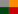IP Subnet Calculator

The IP Subnet Mask Calculator enables subnet network calculations using network class, IP address, subnet mask, subnet bits, mask bits, maximum required IP subnets and maximum required hosts per subnet.

Results of the subnet calculation provide the hexadecimal IP address, the wildcard mask, for use with ACL (Access Control Lists), subnet ID, broadcast address, the subnet address range for the resulting subnet network and a subnet bitmap.

For classless supernetting, please use the CIDR Calculator. For classful supernetting, please use the IP Supernet Calculator. For simple ACL (Access Control List) wildcard mask calculations, please use the ACL Wildcard Mask Calculator.

Note:
These online network calculators may be used totally free of charge provided their use is from this url (www.subnet-calculator.com).Subnet Calculator

## Subnet Bitmap

1. The subnet calculator implements a classful / classed IP addressing scheme where the following rules are adhered to:

• Class A addresses have their first octet in the range 1 to 126 (binary address begins with 0).

• Class B addresses have their first octet in the range 128 to 191 (binary address begins with 10).

• Class C addresses have their first octet in the range 192 to 223 (binary address begins with 110).

2. The subnet calculator allows the use of a single subnet bit - for example, a class C address with a subnet mask of 255.255.255.128 is permitted.

3. The subnet calculator allows a subnet ID to have its final octet equal to the final octet of its subnet mask - for example, a class C network address of 192.168.0.192 with a subnet mask of 255.255.255.192 is permitted.

The above is generally accepted as being 'normal', however, certification students should keep in mind that, in some certification programs, the final two points are regarded as inacceptible.

For classless subnetting, you can use the CIDR calculator.